Courses

# Test: Principle Of Effective Stress, Capillarity & Permeability - 2

## 10 Questions MCQ Test Topicwise Question Bank for GATE Civil Engineering | Test: Principle Of Effective Stress, Capillarity & Permeability - 2

Description
This mock test of Test: Principle Of Effective Stress, Capillarity & Permeability - 2 for Civil Engineering (CE) helps you for every Civil Engineering (CE) entrance exam. This contains 10 Multiple Choice Questions for Civil Engineering (CE) Test: Principle Of Effective Stress, Capillarity & Permeability - 2 (mcq) to study with solutions a complete question bank. The solved questions answers in this Test: Principle Of Effective Stress, Capillarity & Permeability - 2 quiz give you a good mix of easy questions and tough questions. Civil Engineering (CE) students definitely take this Test: Principle Of Effective Stress, Capillarity & Permeability - 2 exercise for a better result in the exam. You can find other Test: Principle Of Effective Stress, Capillarity & Permeability - 2 extra questions, long questions & short questions for Civil Engineering (CE) on EduRev as well by searching above.
QUESTION: 1

Solution:
QUESTION: 2

### In a soil deposit of three layers of equal thickness, the permeabilities of first, second and third layers are in the ratio of 1 : 2 : 3. The ratio of average permeability in horizontal direction (kh) to that in the vertical direction (kv) will be

Solution:

Thickness of each layer is same i.e.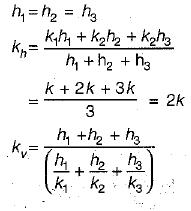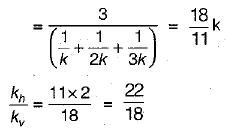QUESTION: 3

### Rise of water table above the ground surface causes:

Solution:

Water table above the ground surface acts as surcharge hence total stress also increases.

Also,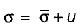and as u increases σ increases

QUESTION: 4

The water level in a lake is 5 m above the bed. The saturated unit weight of the lake bed soil is 20 kN/m3. The unit weight of water is 10 kN/m3. The effective vertical stress at 5 m depth below . the lake bed is

Solution: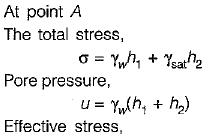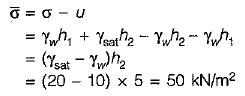QUESTION: 5

Due to rise in temperature, the viscosity and the unit weight of the percolating fluid are reduced to 60% and 90% respectively. If other things remain constant, the coefficient of permeability

Solution: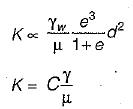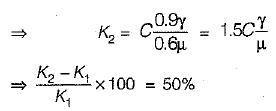QUESTION: 6

Consider the following statements:
Capillary water in soils
1. Causes negative pore water pressure.
2. Reduces effective pressure
3. Increases bearing capacity
4. Reduces bearing capacity

Which of these statements are correct?

Solution:

Capillary water causes negative pore pressure, increases effective pressure and bearing capacity.

QUESTION: 7

Effective stress on soil:

Solution:
QUESTION: 8

In a falling-head permeability test, the time taken for the head to fall from 27 cm to 3 cm is 10 minutes, if the test is repeated with the same initial head, i.e., 27 cm, what time would it take for the head to fall to 9 cm?

Solution: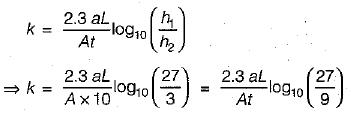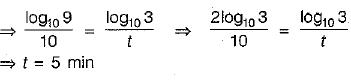QUESTION: 9

The ratio of Darcy’s coefficient of permeability to porosity is known as:

Solution:

Kp = K/n
Kp = Coefficient of percolation

QUESTION: 10

The soils most susceptible to liquefaction are

Solution:

In loose saturated sands on disturbance soil molecules came closer and volume decreases and hence positive pore pressure is set up, as a result effective stress reduces and sudden loss in shear strength is observed which results into failure. Such phenomenon is called liquefaction of sands.